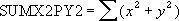## Excel Office

Excel How Tos, Tutorials, Tips & Tricks, Shortcuts

# SUMX2PY2 function: Description, Usage, Syntax, Examples and Explanation

## What is SUMX2PY2 function in Excel?

SUMX2PY2 function is one of the Math and Trig functions in Microsoft Excel that returns the sum of the sum of squares of corresponding values in two arrays. The sum of the sum of squares is a common term in many statistical calculations.

## Syntax of SUMX2PY2 function

SUMX2PY2(array_x, array_y)

The SUMX2PY2 function syntax has the following arguments:

• Array_x: The first array or range of values.
• Array_y: The second array or range of values.

## SUMX2PY2 formula explanation

• The arguments should be either numbers or names, arrays, or references that contain numbers.
• If an array or reference argument contains text, logical values, or empty cells, those values are ignored; however, cells with the value zero are included.
• If array_x and array_y have a different number of values, SUMX2PY2 returns the #N/A error value.
• The equation for the sum of the sum of squares is:## Example of SUMX2PY2 function

Steps to follow:

1. Open a new Excel worksheet.

2. Copy data in the following table below and paste it in cell A1

Note: For formulas to show results, select them, press F2 key on your keyboard and then press Enter.

You can adjust the column widths to see all the data, if need be.

 Data First array Second array 2 6 3 5 9 11 1 7 8 5 7 4 5 4 Formula Description (Result) Result =SUMX2PY2(A3:A9,B3:B9) Sum of the sum of squares of the two arrays above (521) 521 =SUMX2PY2({2,3,9,1,8,7,5}, {6,5,11,7,5,4,4}) Sum of the sum of squares of the two arrays constants (521) 521